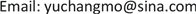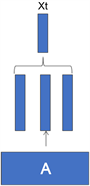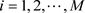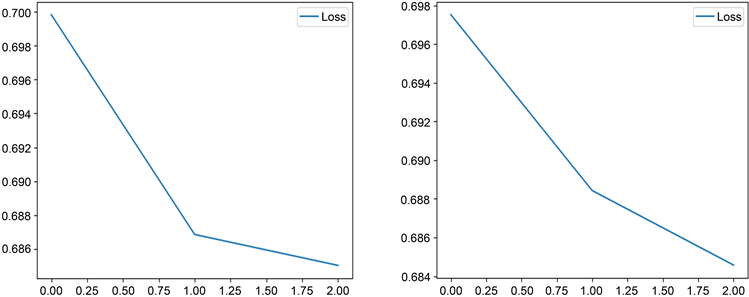﻿ 基于电子病历的疾病风险预测 Disease Risk Prediction Based on EHR

Hans Journal of Data Mining
Vol. 10  No. 01 ( 2020 ), Article ID: 33876 , 9 pages
10.12677/HJDM.2020.101005

Disease Risk Prediction Based on EHR

Ningning Li1, Qian Lu1, Min Liang1, Dong Lin2, Yuchang Mo1

1Fujian Province University Key Laboratory of Computational Science, School of Mathematical Sciences, Huaqiao University, Quanzhou Fujian

2College of Acupuncture, Fujian University of Traditional Chinese Medicine, Fuzhou FujianReceived: Dec. 23rd, 2019; accepted: Jan. 1st, 2020; published: Jan. 8th, 2020ABSTRACT

Data driven health care, as the use of available large-scale medical data, to provide the best and most personalized care, is becoming one of the main trends of the success of the revolution in the medical industry. Electronic health record is the main carrier to promote the success of this data-driven medical revolution. In this paper, we use the method of deep learning, based on the word embedding model to express the EHR information, and use the characteristics of the long-term memory network model to solve the irregular time of EHR information and the long-term dependence of disease information, so as to achieve the prediction of disease risk. Compared with the convolution neural network model, the results show the effectiveness of this method.

Keywords:Deep Learning, Electronic Health Records, Word Embedding, Long Short-Term Memory Network1华侨大学，数学科学学院，计算科学福建省高校重点实验室，福建 泉州

2福建中医药大学，针灸学院，福建 福州1. 引言

2. 相关工作

3. 基本概念

3.1. 词表示

3.2. 学习词嵌入矩阵

$h=\frac{{E}^{{w}_{i-C}}+\cdots +{E}^{{w}_{i-1}}+{E}^{{w}_{i+1}}+\cdots +{E}^{{w}_{i+C}}}{2C}$ (1)

$P\left({w}_{i}|{w}_{i-C},\cdots ,{w}_{i-1},{w}_{i+1},\cdots ,{w}_{i+C}\right)=\text{softmax}\left(a\right)$ (2)

$L=\frac{1}{T}\underset{i=1}{\overset{T}{\sum }}\mathrm{log}P\left({w}_{i}|{w}_{i-C},\cdots ,{w}_{i-1},{w}_{i+1},\cdots ,{w}_{i+C}\right)$ (3)

3.3. 长短期记忆网络(LSTM)

${i}_{t}=\sigma \left({W}_{i}{x}_{t}+{U}_{i}{h}_{t-1}+{b}_{i}\right)$ (4)

${f}_{t}=\sigma \left({W}_{f}{x}_{t}+{U}_{f}{h}_{t-1}+{b}_{f}\right)$ (5)

${o}_{t}=\sigma \left({W}_{o}{x}_{t}+{U}_{o}{h}_{t-1}+{b}_{0}\right)$ (6)

${g}_{t}=\mathrm{tanh}\left({W}_{c}{x}_{t}+{U}_{c}{h}_{t-1}+{b}_{c}\right)$ (7)

${c}_{t}={f}_{t}\ast {c}_{t-1}+{i}_{t}\ast {g}_{t}$ (8)

${h}_{t}={o}_{t}\ast \mathrm{tanh}\left({c}_{t}\right)$ (9)

3.4. 模型复杂性

LSTM层的参数：

4. 基于电子病历的风险预测模型

4.1. 可变大小的入院信息的表示Figure 1. Code embedding

4.2. 池化

${x}_{t}^{i}$ 是向量 ${x}_{t}$ 的第i个元素，入院采用最大池化、合并池化或平均池化，合并方式如下：

${x}_{t}^{i}=\mathrm{max}\left({A}_{i}^{{d}_{1}},{A}_{i}^{{d}_{2}},\cdots ,{A}_{i}^{{d}_{h}}\right)$

$i=1,2,\cdots ,M$。这类似于选择性注意诊断和干预之间的影响最大的因素。它也类似于通常的编码实践，即选择一个诊断作为入院的主要原因。

${x}_{t}^{i}=\frac{{A}_{i}^{{d}_{1}}+{A}_{i}^{{d}_{2}}+\cdots +{A}_{i}^{{d}_{h}}}{\sqrt{|{A}_{i}^{{d}_{1}}+{A}_{i}^{{d}_{2}}+\cdots +{A}_{i}^{{d}_{h}}|}}$。归一化降低了大量的诊断和干预的影响。

${x}_{t}=\frac{{A}^{{d}_{1}}+{A}^{{d}_{2}}+\cdots +{A}^{{d}_{h}}}{h}$

4.3. LSTM预测模型

${\stackrel{^}{y}}_{p}=\text{softmax}\left({W}_{y}{z}^{\left(p\right)}+{b}_{y}\right)$ (10)

$\theta$ 为LSTM模型中所有参数的集合，预测概率向量 ${\stackrel{^}{y}}_{p}$ 也可用模型后验分布 $P\left({y}_{p}|{X}^{p};\theta \right)$ 表示，其中 ${y}_{p}$ 是真值。利用真值 ${y}_{p}$ 与预测概率 ${\stackrel{^}{y}}_{p}$ 之间的交叉熵来计算损失，因此，风险预测的目标函数是交叉熵的平均值：

$L\left(\theta \right)=-\frac{1}{|P|}\underset{p=1}{\overset{|P|}{\sum }}\left({y}_{p}^{T}\mathrm{log}\left({\stackrel{^}{y}}_{p}\right)+{\left(1-{y}_{p}\right)}^{T}\mathrm{log}\left(1-{\stackrel{^}{y}}_{p}\right)\right)$ (11)

5. 实验

5.1. 实验设置Table 1. Parameter settings on the prediction model LSTMTable 2. Parameter settings on prediction model CNN

5.2. 实验结果与分析Figure 2. Loss image of CCN and LSTM modelsTable 4. Performance evaluation of the three models

6. 结论与展望

Disease Risk Prediction Based on EHR[J]. 数据挖掘, 2020, 10(01): 47-55. https://doi.org/10.12677/HJDM.2020.101005

1. 1. Jensen, P.B., Jensen, L.J. and Brunak, S. (2012) Mining Electronic Health Records: Towards Better Research Applica-tions and Clinical Care. Nature Reviews Genetics, 13, 395-405.
https://doi.org/10.1038/nrg3208

2. 2. Caroprese, L., Veltri, P., Vocaturo, E. and Zumpano, E. (2018) Deep Learning Techniques for Electronic Health Record Analysis. 2018 9th International Conference on Information, Intelligence, Systems and Applications, Zakynthos, Greece, 23-25 July 2018, 1-4.
https://doi.org/10.1109/IISA.2018.8633647

3. 3. Madsen, L.B. (2014) Data-Driven Healthcare: How Analytics and BI Are Transforming the Industry. MIT Technology Review Business Report, 117, 1-19.

4. 4. Madsen, L.B. (2014) Data-Driven Healthcare: How Analytics and BI Are Transforming the Industry. Wiley, New York.

5. 5. Ho, J.C., Ghosh, J. and Sun, J.M. (2014) Marble: High-Throughput Phenotyping from Electronic Health Records via Sparse Nonnegative Tensor Factorization. In: Proceedings of the 20th ACM SIGKDD International Conference on Knowledge Discovery and Data Dining (KDD’14), 115-124.

6. 6. Cheng, Y., Wang, F., Zhang, P. and Hu, J.Y. (2016) Risk Pre-diction with Electronic Health Records: A Deep Learning Approach. Proceedings of the 2016 SIAM International Con-ference on Data Mining (SDM’ 16), Miami, FL, 5-7 May 2016, 432-440.
https://doi.org/10.1137/1.9781611974348.49

7. 7. Zhou, J., Wang, F., Hu, J. and Ye, J. (2014) From Micro to Macro: Data Driven Phenotyping by Densification Longitudinal Electronic Medical Records. In: Proceedings of the 20th ACM SIGKDD International Conference on Knowledge Discovery and Data Mining, ACM, New York, 135-144.
https://doi.org/10.1145/2623330.2623711

8. 8. Wang, F., Zhang, P., Qian, B., Wang, X. and Davidson, I. (2014) Clinical Risk Prediction with Multilinear Sparse Logistic Regression. In: Proceedings of the 20th ACM SIGKDD Inter-national Conference on Knowledge Discovery and Data mining, ACM, New York, 145-154.
https://doi.org/10.1145/2623330.2623755

9. 9. Chen, L. (2019) Assertion Detection in Clinical Natural Language Pro-cessing: A Knowledge-Poor Machine Learning Approach. 2019 IEEE 2nd International Conference on Information and Computer Technologies, Kahului, HI, 14-17 March 2019, 37-40.
https://doi.org/10.1109/INFOCT.2019.8710921

10. 10. Hochreiter, S. and Schmidhuber, J. (1997) Long Short-Term Memory. Neural Computation, 9, 1735-1780.
https://doi.org/10.1162/neco.1997.9.8.1735

11. 11. Rumelhart, D.E. (1986) Learning Representations by Back-Propagating Errors. Nature, 323, 533-536.
https://doi.org/10.1038/323533a0

12. 12. Choi, E., Bahadori, M.T., Searles, E., et al. (2016) Multi-Layer Representa-tion Learning for Medical Concepts. Proceedings of the 22nd ACM SIGKDD International Conference on Knowledge Discovery and Data Mining, August 2016, 1495-1504.
https://doi.org/10.1145/2939672.2939823

13. 13. Choi, E., Bahadori, M., Song, L., et al. (2016) GRAM: Graph-Based Attention Model for Healthcare Representation Learning. Computer Science, 787-795.

14. 14. Ma, F.L., Radha, C. and Zhou, J. (2017) Dipole: Diagnosis Prediction in Healthcare via Attention-based Bidirectional Recurrent Neural Networks. Proceedings of the 23rd ACM SIGKDD International Conference on Knowledge Discovery and Data Mining, August 2017, 1903-1911.
https://doi.org/10.1145/3097983.3098088

15. 15. Che, Z., Cheng, Y., Zhai, S., Sun, Z. and Liu, Y. (2017) Boosting Deep Learning Risk Prediction with Generative Adversarial Networks for Electronic Health Records. 2017 IEEE Inter-national Conference on Data Mining, New Orleans, LA, 18-21 November 2017, 787-792.
https://doi.org/10.1109/ICDM.2017.93

16. 16. Choi, E., Bahadori, M.T., Kulas, J.A., Schuetz, A. and Stewart, W.F. (2016) RETAIN: An Interpretable Predictive Model for Healthcare Using Reverse Time Attention Mechanism. Computer Science, 3504-3512.

17. 17. Suo, Q., Ma, F., Yuan, Y., et al. (2017) Personalized Disease Prediction Using a CNN-Based Similarity Learning Method. 2017 IEEE International Conference on Bioinformatics and Biomedicine, Kansas City, MO, 13-16 November 2017, 811-816.
https://doi.org/10.1109/BIBM.2017.8217759